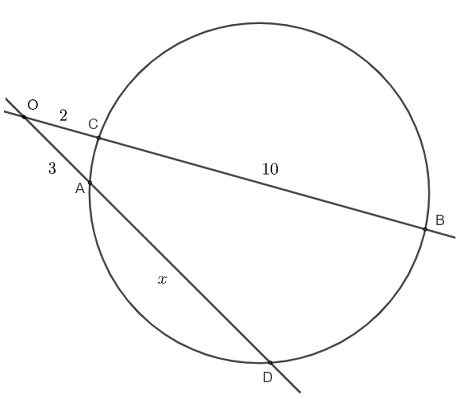# Intersecting Secant Theorem Questions with Solutions

Consider the circle and the secants $A B$ and $C D$ such that each cuts the circle at two points.The intersecting secant theorem  states that for the two secant $A B$ and $C D$ intersecting a circle, there is relationship between the lengths of the segments as follows: $OA \times OB = OC \times OD$

## Question With Solutions

Question 1
Given the lengths of segments: $OA = 3 , OC = 2$ and $CB = 10$, Find $x = AD$ in the figure below.Solution
Apply the intersecting secant theorem above to the secant $OD$ and $OB$ to write: $\quad OA \times OD = OC \times OB$
Substitute the known and given quantities: $\quad 3 \times (3+x) = 2 \times (2+10)$
Expand and simplify: $\quad 9 + 3 x = 24$
Solve for $x$: $\quad x = \dfrac{24-9}{3} = 5$

Question 2
Given the lengths of segments $OA = x , OC = 3, CD = 13$ and $AB = 2x$, find $x$.Solution
Apply the intersecting secant theorem to $OB$ and $OD$ to write: $\quad OA \times OB = OC \times OD$
Substitute the given quantities: $\quad x \times (x + 2x) = 3 \times (3+13)$
Expand and group like terms: $\quad 3 x^2 = 48$
Divide both sides by $3$ and rewrite the above equation as: $x^2 = 16$
Solve for $x$: $\quad x = \pm 4$
$x$ must be positive because the lengths of segments are positive, hence the solution to the given question is: $x = 4$

Question 3
In the figure below, $OB$ and $OD$ are secant to the circle such that $OD$ passes through the center $G$ of the circle and $OA = 4, AB = 10$ and $OC = 3$. Find the length of the radius of the circle.Solution
Since $G$ is the center of the circle, $CD$ is a diameter and $CD = 2 r$ where $r$ is the length of the radius.
Apply the intersecting secant theorem to $OB$ and $OD$ to write: $\quad OA \times OB = OC \times OD$
Substitute the known and unknown quantities: $\quad 4 \times (4 + 10) = 3 \times (3+2r)$
Expand: $\quad 56 = 9+6r$
Group and solve for $r$: $\quad r = \dfrac{47}{6}$

Question 4
In the figure below, $OB$ and $OD$ are secant to the circle. Find the relationship between $x$ and $y$ of the form $a x + b y = c$ such that in the figure below, $OC = 45 , OA = 42, CD = y$ and $AB = x$.Solution
The intersecting secant theorem to $OB$ and $OD$ to write: $\quad OA \times OB = OC \times OD$
Substitute the known quantities: $\quad 42 \times (42 + x) = 45 \times (45 + y)$
Expand: $\quad 1764 + 42 x = 2025 + 45y$
The above may be written as: $\quad 42 x - 45 y = 261$